# How to exit an if statement in Python [5 Ways]Last updated: Aug 27, 2023
4 min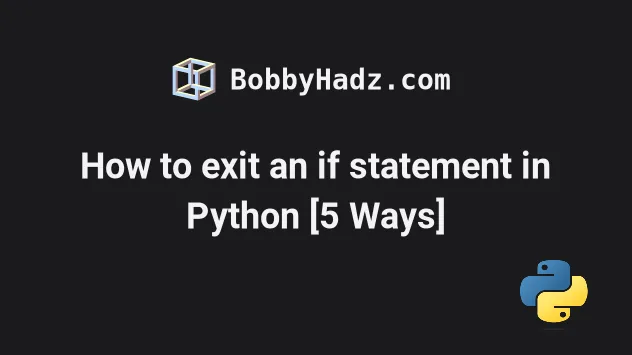## #How to exit an if statement in Python

There are multiple ways to exit an `if` statement in Python:

• Use `return` to exit an `if` statement in a function.
• Use `break` to exit an if statement in a for or a while loop.
• Use `try/except` to exit an `if` statement by throwing an error.
• Use `if/elif` to check for multiple conditions.
• Use `sys.exit` if you want to raise a `SystemExit` exception.

## #Exiting an `if` statement in a function

If you need to exit an `if` statement in a function, use the `return` statement.

main.py
```Copied!```def my_function(num):
if num > 0:
print('num > 0')
return
if num < 0:
print('num < 0')
return

print('num = 0')
return

my_function(100) # 👉️ num > 0

print('-' * 50)

my_function(-30) # 👉️ num < 0

print('-' * 50)

my_function(0) # 👉️ num = 0
``````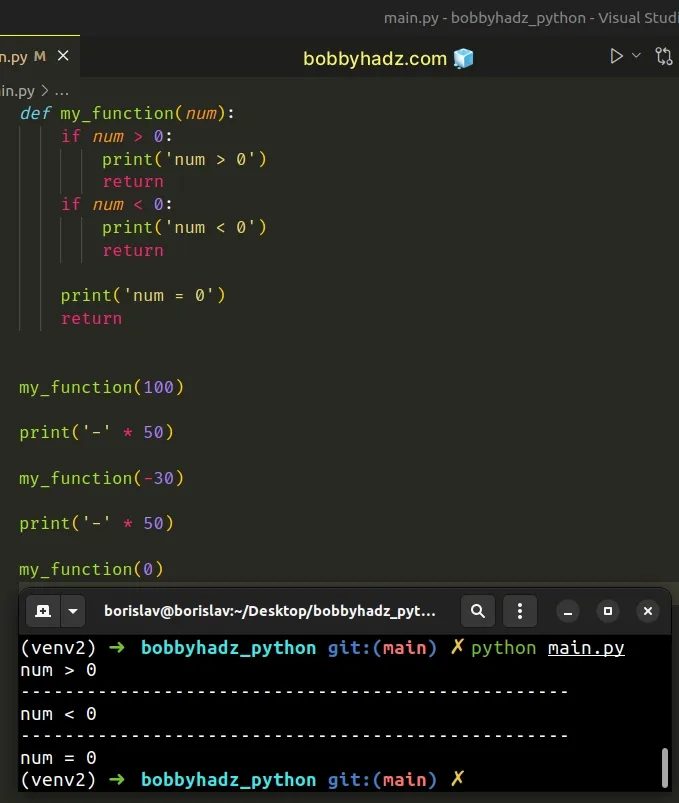The `return` statement enables you to exit a function by returning a specific value.

Returning a value is not necessary.

If you `return` nothing, the function returns `None`.

However, you could also return a value in the `if` block that is exited.

main.py
```Copied!```def my_function(num):
if num > 0:
print('num > 0')
return 'A'
if num < 0:
print('num < 0')
return 'B'

print('num = 0')
return 'C'

result = my_function(100)
print(result)  # 👉️ A

print('-' * 50)

result = my_function(-30)
print(result)  # 👉️ B

print('-' * 50)

result = my_function(0)
print(result)  # 👉️ C
``````

Running the code sample produces the following output.

shell
```Copied!```def my_function(num):
if num > 0:
print('num > 0')
return 'A'
if num < 0:
print('num < 0')
return 'B'

print('num = 0')
return 'C'

result = my_function(100)
print(result)  # 👉️ A

print('-' * 50)

result = my_function(-30)
print(result)  # 👉️ B

print('-' * 50)

result = my_function(0)
print(result)  # 👉️ C
``````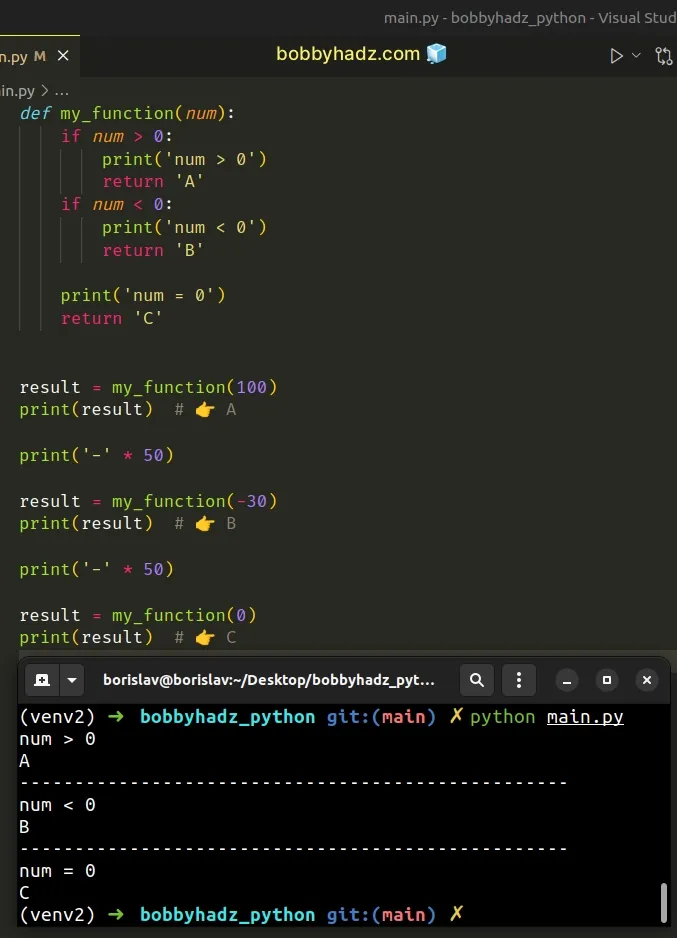The function in the example exits the `if` statements by returning a specific value.

Once the `return` statement runs, the Python interpreter exits the `if` block (and the function) and continues parsing the rest of your code.

## #Use `break` to exit an if statement in a for or a while loop

If you need to exit an `if` statement in a for loop or a `while` loop, use the break statement.

main.py
```Copied!```for i in range(1, 7):
print(i)

if i == 3:
print(i)
break
if i == 6:
print(i)
break
``````

Running the code sample produces the following output.

shell
```Copied!```1
2
3
3
``````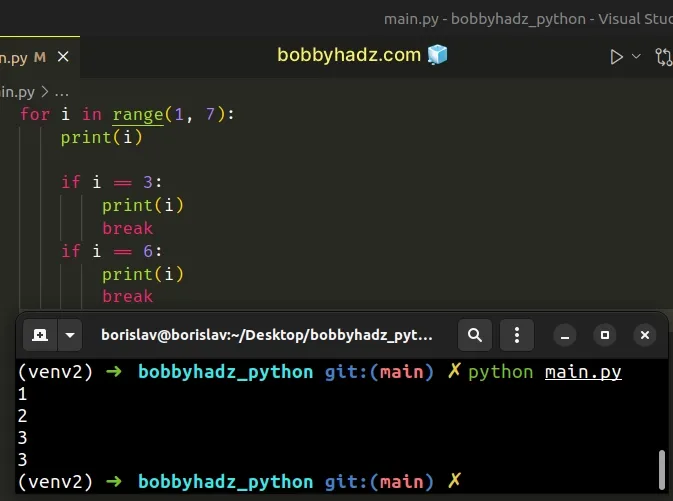The `break` statement breaks out of the innermost enclosing `for` or `while` loop.

The code sample uses the range class to iterate over an object containing the numbers from `1` to `6`.

main.py
```Copied!```# [1, 2, 3, 4, 5, 6]
print(list(range(1, 7)))
``````

On each iteration, we check if the current number is equal to `3`.

main.py
```Copied!```for i in range(1, 7):
print(i)

if i == 3:
print(i)
break
if i == 6:
print(i)
break
``````

If the condition is met, we use the `break` statement to exit the `if` statement and the `for` loop.

You can also use the `break` statement to exit an `if` statement in a `while` loop.

main.py
```Copied!```num = 6

while num > 0:
if num % 2 == 0:
num = num - 10
break
if num % 3 == 0:
num = num - 20
break
break

print(num)  # 👉️ -4
``````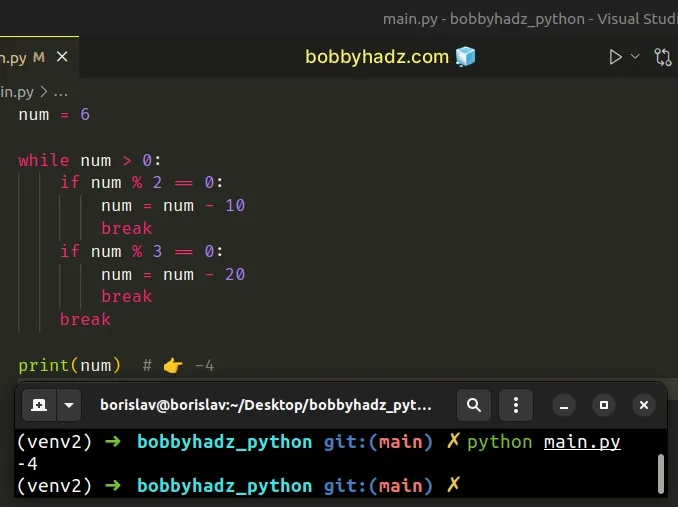The first `if` statement checks if the `num` variable is divisible by `2`.

If the condition is met, we subtract `10` from the `num` variable and exit the `if` statement and the `while` loop with the `break` keyword.

The code sample returns `-4` because `6 - 10 = -4`.

## #Use `try/except` to exit an `if` statement by throwing an error

You can also exit an `if` statement by throwing an error with the `raise` keyword.

main.py
```Copied!```
num = 6

try:
if num % 2 == 0:
print('Number is divisible by 2')
raise ValueError('some error')

print('This will not run')
except ValueError:
print('Except block runs')
``````

Running the code sample produces the following output.

shell
```Copied!```Number is divisible by 2

Except block runs
``````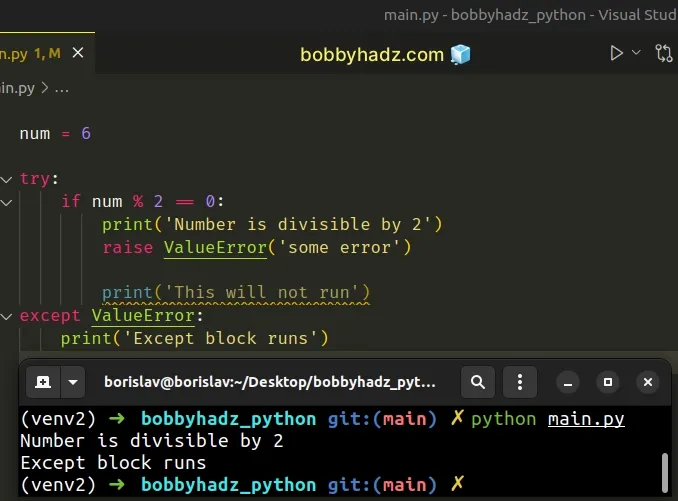The line after the `raise ValueError` statement doesn't run.

Instead, the raised exception gets handled by the `except` block.

You can also use the pass statement.

main.py
```Copied!```num = 6

try:
if num % 2 == 0:
print('Number is divisible by 2')
raise ValueError('some error')

print('This will not run')
except ValueError:
pass
``````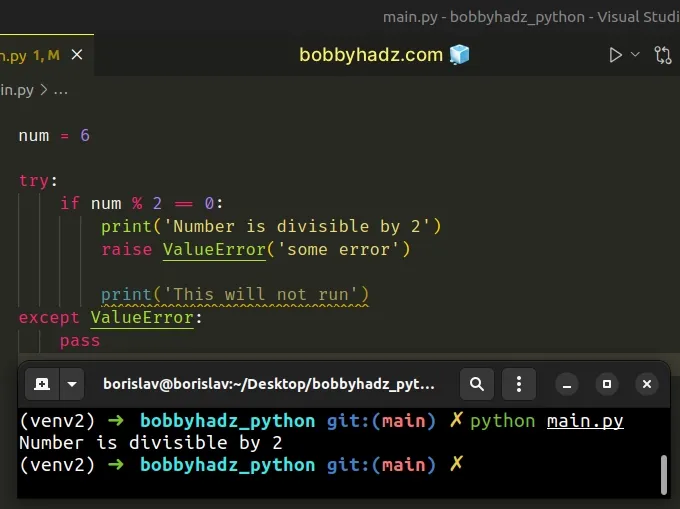The `pass` statement does nothing and is used when a statement is required syntactically but the program requires no action.

## #Using `if/elif/else` statements to check for multiple conditions

If you need to check for multiple conditions in separate conditional statements, use `if/elif` blocks with an optional `else`.

main.py
```Copied!```num = 9

if num > 10:
print('num > 10')
elif num > 9:
print('num > 9')
elif num > 8:
# 👇️ this runs
print('num > 8')
else:
print('else block runs')
``````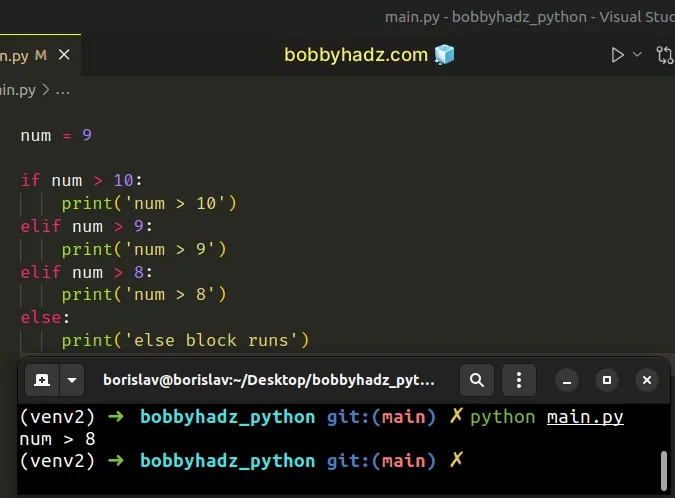The `if` statement checks if the `num` variable stores a value that is greater than `10`.

The `elif` statements are only checked if the condition in the `if` statement evaluates to `False`.

If the `if` statement evaluates to `True`, the `elif` and `else` statements won't run.

If either of the `elif` statements evaluates to `True`, the corresponding block runs and the subsequent statements are not checked.

The `else` block only runs if neither of the `if` and `elif` statements evaluate to `True`.

## #Using the `sys.exit()` method to exit an `if` statement

The sys.exit method is used to signal an intention to exit the interpreter.

Using this approach is generally not recommended because most of the time there are better ways to achieve the same result.

main.py
```Copied!```import sys

num = 9

if num == 9:
print('num > 10')

sys.exit()

print('This never runs')
``````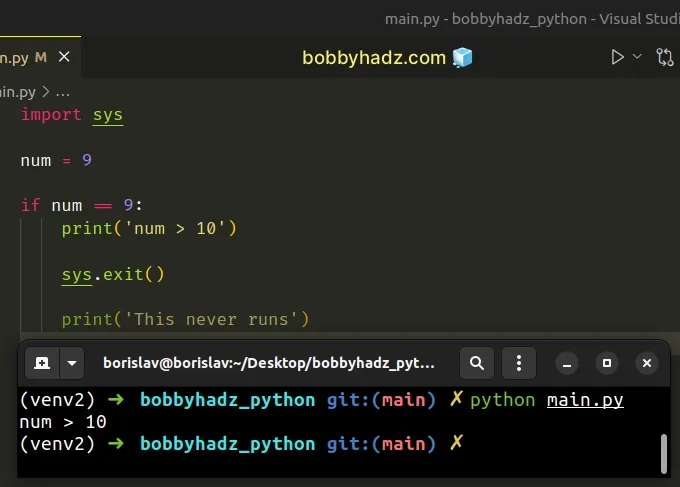The lines after the `sys.exit()` method call in the `if` statement will never run.